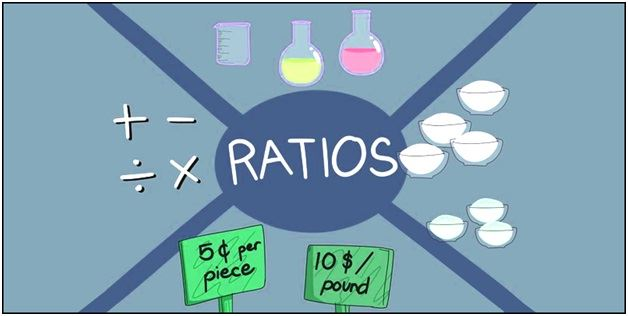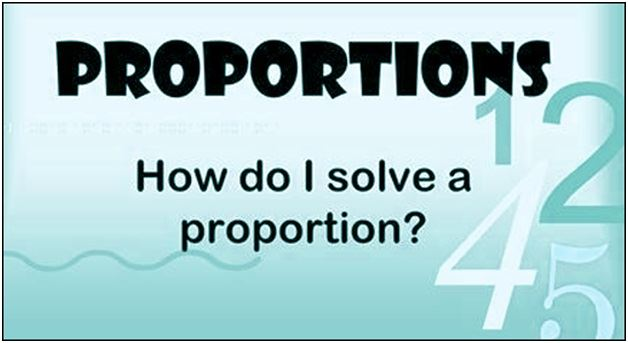# Ratios And Proportion

The ratio and proportion are one of the most essential concepts in maths. The concept of ratio defines us to compare two quantities while the proportion is an equation which shows that two ratios are equivalent. Hence, it is easy to calculate the ratio of the given proportion.

Suppose a and b are two different numbers or integers, then the ratio of these two integers can be represented as a/b or a:b. Whereas, the proportion, on the other hand, is the relation between two ratios such as a:b::c:d or a/b = c/d, where a,b,c and d are integers.

In this article, we are going to discuss all the concepts such as definition, ratio and proportion formulas, and examples in detail.

## Ratio and Proportion Definition

The definition of ratio and proportion is described here in this section. Both concepts are an important part of Mathematics. In real life also, you may find a lot of examples such as the rate of speed (distance/time) or price rate (rupees/meter) of a material, etc, where the concept of the ratio is highlighted.

Whereas, the proportion explains where the two ratios are equivalent to each other. For example, the time taken by train to cover 100km per hour is equal to the time taken by it to cover the distance of 500km for 5 hours. Such as 100km/hr = 500km/5hrs = 100km/hr.

Let us now learn both the concept one by one.

### What is the ratio?

In certain circumstances, the comparison by the process of division makes good sense when compared to performing their difference. Hence, the comparison of two quantities by the process of division method is called as ‘Ratio’ between two numbers.The two numbers in a ratio can only be compared when they have the same unit. We make use of ratios to compare two things. The sign used to denote a ratio is ‘:’.

A ratio can be written as a fraction, say 2/5. Or can write it by using “to”, as “2 to 5.” We happen to see various comparisons or say ratios in our daily life.

### What is the proportion?

Ratio and proportions are said to be faces of the same coin. When two ratios are equal in value, then they are said to be in proportion. In simple words, it compares two ratios. Proportions are denoted by the symbol  ‘::’ or ‘=’.### Continued Proportion

Consider two ratios to be a: b and c: d.

Then in order to find the continued proportion for the two given ratio terms, we convert the means to a single term/number. This would, in general, be the LCM of means.

For the given ratio, the LCM of b & c will be bc.

Thus, multiplying the first ratio by c and second ratio by b, we have

First ratio- ca:bc

Second ratio- bc: bd

Thus, the continued proportion can be written in the form of ca: bc: bd

## Ratio and Proportion Formula

Suppose we have two quantities or two numbers or two entities and we have to find the ratio of these two, then the formula for ratio is defined as;

a: b ⇒ a/b

where a and b could be any two quantities.

Here, “a” is called the first term or antecedent, and “b” is called the second term or consequent.

Now, let us assume that, in proportion, the two ratios are a:b & c:d. The two terms ‘b’ and ‘c’ are called ‘means or mean term,’ whereas the terms ‘a’ and ‘d’ are known as ‘extremes or extreme terms.’

 a/b = c/d or  a : b :: c : d

Let us consider one more example of a number of students in a classroom. Our first ratio of the number of girls to boys is 3:5 and that of the other is 4:8, then the proportion can be written as

3 : 5 ::  4 : 8 or 3/5 = 4/8

Here 3 & 8 are the extremes, while 5 & 4 are the means.

Note: The ratio value does not affect when the same non-zero number is multiplied or divided on each term.

### Ratio and Proportion Tricks

Let us learn here some rules and tricks to solve problems based on ratio and proportion topic.

• If u/v = x/y, then uy = vx
• If u/v = x/y, then u/x = v/y
• If u/v = x/y, then v/u = y/x
• If u/v = x/y, then (u+v)/v = (x+y)/y
• If u/v = x/y, then (u-v)/v = (x-y)/y
• If u/v = x/y, then (u+v)/ (u-v) = (x+y)/(x-y), which is known as componendo -Dividendo Rule
• If u/v = v/x, then u/x = u2/v2
• If u/v = x/y, then u = x and v =y
• If a/(b+c) = b/(c+a) = c/(a+b) and a+b+ c ≠0, then a =b = c

## Ratio and Proportion Examples

Question 1: Are the ratios 4:5 and 8:10 said to be in Proportion?

Solution:

4:5= 4/5 = 0.8 and 8: 10= 8/10= 0.8

Since both the ratios are equal, they are said to be in proportion.

Question 2: Are the two ratios 8:10 and 7:10 in proportion?

Solution:

8:10= 8/10= 0.8 and 7:10= 7/10= 0.7

Since both the ratios are not equal, they are not in proportion.

Question 3: Given ratio are-

a:b = 2:3

b:c = 5:2

c:d = 1:4

Find a: b: c.

Solution:

Multiplying the first ratio by 5, second by 3 and third by 6, we have

a:b = 10: 15

b:c = 15 : 6

c:d = 6 : 24

In the ratio’s above, all the mean terms are equal, thus

a:b:c:d = 10:15:6:24

### Ratio and Proportion Word Problems

Question 4Out of the total students in a class, if the number of boys is 5 and the number of girls being 3, then find the ratio between girls and boys.

Solution: The ratio between girls and boys can be written as 3:5( Girls: Boys). The ratio can also be written in the form of factor like 3/5.

Question 5: Two numbers are in the ratio 2 : 3. If the sum of numbers is 60, find the numbers.

Solution: Given, 2/3 is the ratio of any two numbers.

Let the two numbers be 2x and 3x.

As per the given question, the sum of these two numbers = 60

So, 2x + 3x = 60

5x = 60

x = 12

Hence, the two numbers are;

2x = 2 x 12 = 24

and

3x = 3 x 12 = 36

24 and 36 are the required numbers.

Likewise, ratio and proportion are used to solve many real-world problems. Register with BYJU’S and get solutions for many difficult questions in easy and followed by the step-by-step procedure.